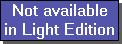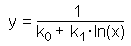The SDL Component Suite is an industry leading collection of components supporting scientific and engineering computing. Please visit the SDL Web site for more information....## CalcReciLogFitUnit: SDL_math2 Class: Declaration: procedure CalcReciLogFit (var k0, k1, FitQual: double);

The procedure CalcReciLogFit calculates the best fitting reciprocal logarithmic curve for a given set of data. The curve is determined by the equationThe values of x and y are given by the data samples, the parameters k0 and k1 are estimated by CalcReciLogFit using a least squares approximation.

The data points [x,y] have to be entered using the routine EnterStatValue. A minimum number of 3 values is required in order to apply CalcReciLogFit. Do not forget to reset the statistics calculation before entering any new data sets (use the method Init)

In addition to the parameters k0 and k1 CalcReciLogFit the quality of fit FitQual. This parameter may vary between 0.0 and 1.0, indicating the best possible fit if FitQual equals 1.0.

 Hint 1: The quality of fit calculated by CalcReciLogFit is not adjusted for the degree of freedoms in the regression parameters.

 Hint 2: Both the x- and the y-values must be greater than zero. The curve fitting algorithm raises an exception in the case of negative or zero values.

 Example: This method is used in the following example program (see http://www.lohninger.com/examples.html for downloading the code): curvefit

Last Update: 2012-Oct-20